Slider

# Employment/Purpose

A slider component represents a slider with a scale and a knob. It can be used to let user select a value by sliding the knob along the scale. A slider accepts a range of value starting from 0 to certain maximum value. The default maximum value of slider scale is 100. You could change the maximum allowed value by the `maxpos` property. However the default minimum is 0 and cannot be changed.

# Example

```  <slider id="slider" orient="vertical"/>
<slider curpos="1" maxpos="20"  />
```

# Minimal Position

```Since  7.0.1
```

Slider supports minimal position, which can be changed by the minpos property as follows.

```  <slider minpos="30"/>
```

Slider also provides `setRange(int, int)` and `setRange(double, double)` methods to help user change the range from minimal position to maximum position.

# Decimal Mode

```Since  7.0.1
```

Set the mode property to "decimal" will enable decimal slider. So the slider can represent decimal number.

```  <slider mode="decimal" step="0.1"/>
```

# Page Increment

By default, the slider will move to the position of the try on which an user clicks. If you prefer to move in a fixed amount (like the scrollbar does), you could specify the amount of value to move by use of Slider.setPageIncrement(int).

```  <slider pageIncrement="10"/>
```

# Step

```Since  7.0.1
```

By default, the slider will scroll to the position continuously when an user drags it. If you prefer to scroll a discrete fixed amount on each step, you can set the amount of value of the `step` property. Step property is useful in decimal mode, slider's position value could be rounded to a fixed number by specifying step property. For example, if we want to retrieve the value from decimal slider in the range of 10.0 to 12.0. As the below picture shows, the default decimal show the value contains only one digit in fractional part.

If we want to retrieve the value which contains two digits in fractional part, we can set the step value to 0.01. Then the value will increase as 10.01, 10.02, 10.02 on each step when scrolling the slider. If step is 0.05, the value will increase as 10.05, 10.10, 10.15 on each step, as below.

```  <slider mode="decimal" minpos="10.0" maxpos="12.0" step="0.05"/>
```

# Knob Mold

• Available for ZK:
•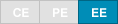Since 8.6.0 Set the mold property to "knob" will enable knob slider. So the slider can act as a normal knob. The knob can controlled by wheel, drag, click and enter a value to the input element.

```  <slider mold="knob" minpos="0.0" maxpos="100.0" curpos="80.5" step="0.5" strokeWidth="40"/>
```

## AngelArc, StrokeWidth and ScaleInput

AngelArc, strokeWidth and scaleInput are properties only for knob mold. Set the angelArc property with a double for the angle of the knob slider. Set the strokeWidth property with a double for the stroke width of the knob. ScaleInput is the scale ratio of the input size.

```  <slider mold="knob" strokeWidth="40" angelArc="270" scaleInput="1.3" minpos="0.0" maxpos="100.0" curpos="80.5" step="0.5"/>
```

# Supported Events

Name
Event Type
onScroll
Event: ScrollEvent

Denotes the content of a scrollable component has been scrolled by the user.

onScrolling
Event: ScrollEvent

Denotes that the user is scrolling a scrollable component. Notice that the component's content (at the server) won't be changed until onScroll is received. Thus, you have to invoke the `getPos` method in the ScrollEvent class to retrieve the temporary position.

# Supported Molds

Available molds of a component are defined in lang.xml embedded in zul.jar.

Name
Snapshot
default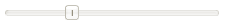sphere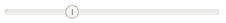scale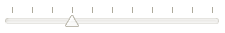Since 8.6.0
knob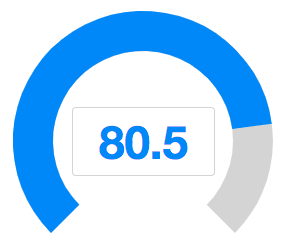Since 7.0.0 the scale mold is deprecated because designs are changed.

```*None
```

# Use Cases

Version Description Example Location

# Version History

Last Update : 2022/01/17

Version Date Content
5.0.4 August 2010 Slider.setPageIncrement(int) is supported.
5.0.4 August 2010 Slider support for clicking to increment or decrement
7.0.1 January 2014 Slider support minimal position and decimal mode

Last Update : 2022/01/17